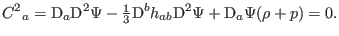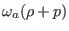### 5.2.2 Anti-Newtonian Limit

We may consider a gravitomagnetic model whose action propagates at infinite speed. Let us define the anti-Newtonian potential as(86)

We substitute Eqs. (85) and (86) into Eq. (77b):(87)

In a spatial infinity, we derive the Helmholtz equation:(88)

Eq. (77b) associates the gravitomagnetic with the angular momentum.

Ashkbiz Danehkar
2018-03-26